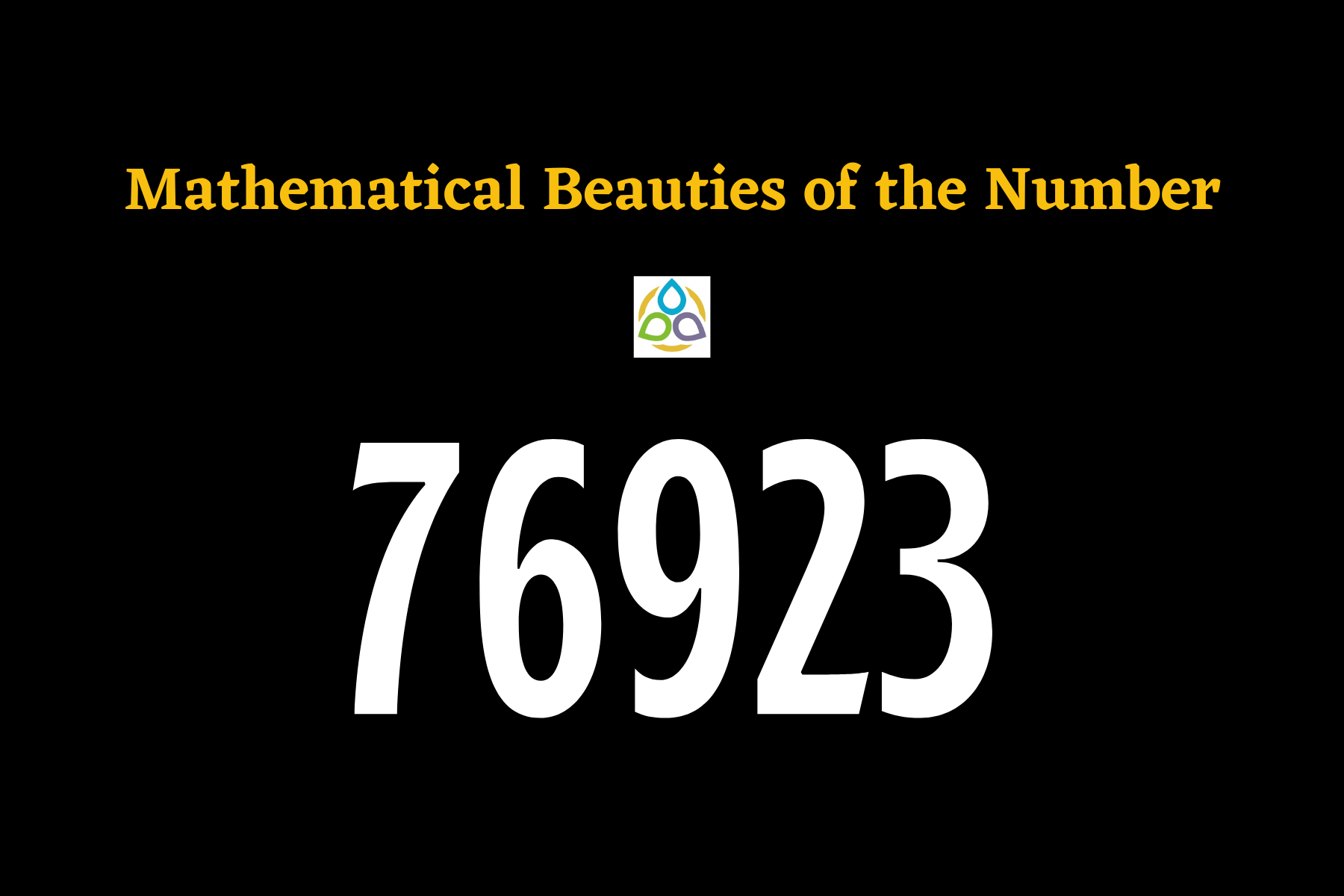# Mathematical Beauties of the Number 76923

Cartesian mathematicism: The long concatenations of simple and easy reasoning which geometricians use in achieving their most difficult demonstrations gave me occasion to imagine that all matters which may enter the human mind were interrelated in the same fashion.
René Descartes

Welcome to the blog Math1089 – Mathematics for All.

I’m glad you came by. I wanted to let you know I appreciate your spending time here on the blog very much. I do appreciate your taking time out of your busy schedule to check out Math1089!

Numbers are always beautiful and in this blog post let’s play with the beautiful number 76923! One of the occurrences of this number can be found in onethirteenth (1/13). Let’s start this post with Midy’s theorem and discuss other interesting properties of the number in succession.

Midy’s Theorem If the period of a repeating decimal for a/p, where p is prime and a/p is a reduced fraction, has an even number of digits, then dividing the repeating portion into halves and adding gives a string of 9s. In the case of 1/13 (onethirteenth), the period is 6. In fact, we have

In this case, we have 076 + 923 = 999.

The prime factorisation of 76923 is 33 × 7 × 11 × 37.

Divisors of 76923 are 1, 3, 7, 9, 11, 21, 27, 33, 37, 63, 77, 99, 111, 189, 231, 259, 297, 333, 407, 693, 777, 999, 1221, 2079, 2331, 2849, 3663, 6993, 8547, 10989, 25641, and 76923.

76923 is a Harshad number as it is divisible by the sum of its digits:

When we multiply 76923 by other numbers, notice how it yields numbers in the same order but with a different starting point. In fact, the first digit of every product goes to the end of the number to form the next product. Otherwise, the order of the digits is intact. Moreover, the sum of the digits in each of these product numbers is equal to 27.

Next, notice how various products of 76,923 yield different numbers. Again, the first digit of the product goes to the end of the number to form the next product. Otherwise, the order of the digits is intact. As before, the sum of the digits in each of these product numbers is equal to 27.

In the above multiplication tables (blue and green), we can see the positive integers from 1 to 12. In fact, it partitions the positive integers from 1 to 12 to into two sets: {1, 3, 4, 9, 10, 12} (first set, blue colour) and {2, 5, 6, 7, 8, 11} (second set, green colour). Is there anything interesting about this division?

We can show that, 6 is the smallest value of n such that 10n – 1 is divisible by 13. In that case, (106 – 1)/13 = 76923 and we get

Your suggestions are eagerly and respectfully welcome! See you soon with a new mathematics blog that you and I call Math1089 – Mathematics for All!“.

1.Biswanath Basu says:

Notes on 76923 are very interesting. Like to follow the blog.

1.Math1089 says:

Thank you so much. Great to hear this.

2.Satyam Pandey says:

That’s amazing effort ❣️
We are motivated from this blogs

1.Math1089 says:

Thank you so much